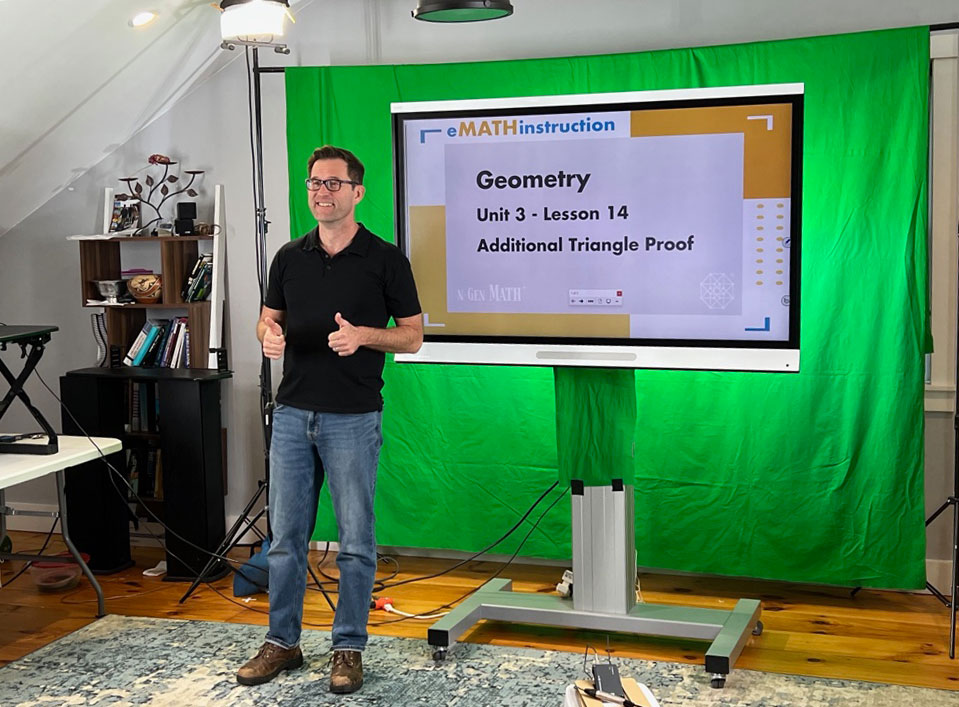Posted onAutumn is beginning to feel far too much like winter here in New York. The leaves are gone from the trees and the weather has turned colder, but here at eMATHinstruction we are heating up with new additions to our materials AND new courses on the way…

### NEW MATERIALS FOR TEACHERS

First, here are the new teacher tools to use in the classroom for each of our courses. A note about our unit assessments: We design all forms of any given unit assessment to test the same standards at the same difficulty level so that teachers can use them as make-up tests or remediation tests:

• Unit 5 (Algebraic Expressions) Form B Unit Assessment
• A Unit 5 practice sheet of problems on evaluating algebraic expressions through substitution. This worksheet gives students a good chance to evaluate simple expressions using order of operations.
• Unit 6 (Linear Equations and Inequalities) Form B Unit Assessment
• A Unit 6 practice set of problems on solving two-step equations — these have integer solutions as well as solutions that are fractional or decimal.
• Unit 4 (Similarity and Dilations) Form B Unit Assessment
• A Unit 4 practice set of problems on dilations in the coordinate plane. In this worksheet, students get practice with plotting dilated figures in the coordinate plane and in identifying/calculating the scaling constant for a particular dilation.
• Unit 5 (Linear Systems) Exit Tickets
• Unit 5 Form A Unit Assessment
• A Unit 5 add-on where students create and then use linear models for populations that are changing at constant rates. We love these longer modeling problems as they give kids a chance to explore and use their math skills in ways that the standard lesson format cannot.
• Unit 4 (Constructions) Mid-Unit Quiz Form D
• A practice set of problems on the circumscribed and inscribed circles of a triangle. These are challenging constructions for students and extra practice is always helpful.
• Unit 5 (Sequences and Series) practice set of problems on arithmetic and geometric series . This is a great set of problems that challenges students to not just use the summation formulas, but to also determine the number of terms in a given series.
• Unit 6 (Quadratic Functions and Their Algebra) Mid-Unit Quiz Form D
• A Unit 6 practice set of problems on solving quadratic systems algebraically. These systems include lines and parabolas, lines and circles, and parabolas and circles. This is a great set of problems to practice some quadratic equation solving.

### N-GEN MATH GEOMETRY IS COMING!

As we move into the winter and out of conference season, we are in the process of finalizing our new N-Gen Math Geometry course. Videos are over half done for the course and the text is in its final editing phase. Just as with N-Gen Math Algebra I, we feel that this course will be an improvement of our Common Core Geometry course. Once it is finished, our full attention will turn to the final book in the series, N-Gen Math Algebra II. The N-Gen Math Geometry book will be available to order next summer for the 2023/2024 school year.

### OUR STANDARDS ALIGNMENT TOOL NOW INCLUDES N-GEN MATH ALGEBRA I!

N-Gen Math Algebra I is now up on EdGate’s alignment tool! Every state has its own math standards. So to be sure our eMATHinstruction materials — including N-Gen Math Algebra I — meet your state’s math standards, use our handy Standards Alignment Tool! Here’s how (for optimal visuals, watch full screen):

Finally, we want to wish everyone a happy and restful Thanksgiving break. Enjoy time with good family, good food and good math.